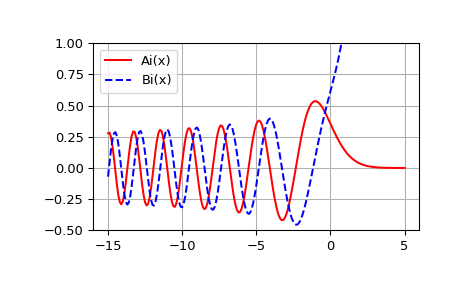# scipy.special.airy¶

scipy.special.airy(z) = <ufunc 'airy'>

Airy functions and their derivatives.

Parameters
zarray_like

Real or complex argument.

Returns
Ai, Aip, Bi, Bipndarrays

Airy functions Ai and Bi, and their derivatives Aip and Bip.

See also

airye

exponentially scaled Airy functions.

Notes

The Airy functions Ai and Bi are two independent solutions of

$y''(x) = x y(x).$

For real z in [-10, 10], the computation is carried out by calling the Cephes  airy routine, which uses power series summation for small z and rational minimax approximations for large z.

Outside this range, the AMOS  zairy and zbiry routines are employed. They are computed using power series for $$|z| < 1$$ and the following relations to modified Bessel functions for larger z (where $$t \equiv 2 z^{3/2}/3$$):

\begin{align}\begin{aligned}Ai(z) = \frac{1}{\pi \sqrt{3}} K_{1/3}(t)\\Ai'(z) = -\frac{z}{\pi \sqrt{3}} K_{2/3}(t)\\Bi(z) = \sqrt{\frac{z}{3}} \left(I_{-1/3}(t) + I_{1/3}(t) \right)\\Bi'(z) = \frac{z}{\sqrt{3}} \left(I_{-2/3}(t) + I_{2/3}(t)\right)\end{aligned}\end{align}

References

1

Cephes Mathematical Functions Library, http://www.netlib.org/cephes/

2

Donald E. Amos, “AMOS, A Portable Package for Bessel Functions of a Complex Argument and Nonnegative Order”, http://netlib.org/amos/

Examples

Compute the Airy functions on the interval [-15, 5].

>>> from scipy import special
>>> x = np.linspace(-15, 5, 201)
>>> ai, aip, bi, bip = special.airy(x)


Plot Ai(x) and Bi(x).

>>> import matplotlib.pyplot as plt
>>> plt.plot(x, ai, 'r', label='Ai(x)')
>>> plt.plot(x, bi, 'b--', label='Bi(x)')
>>> plt.ylim(-0.5, 1.0)
>>> plt.grid()
>>> plt.legend(loc='upper left')
>>> plt.show()#### Previous topic

scipy.special.SpecialFunctionError

#### Next topic

scipy.special.airye